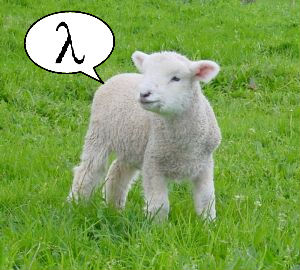# Useful Lambda Expressions

A really useful built in feature of .Net is the Lambda expression. I've been using these for a while, but until I started looking into it, I never realized how useful these can actually be. There are loads of quick, nifty ways to get things done that might take twice as long if not using a lambda expression.

All lambda expressions use the lambda operator =>, which is read as "goes to". Sometimes these expressions look a little confusing, but once you understand the general use they are quite easy to read.Lets take a look at how we traditionally found an item in a generic list:

List<Car> carColours = new List();

``````foreach (Car car in carColours)
{

if (car.Colour == "red")
{
return car.Colour;
}
}
``````

The above code works, but lets try and make this sexy with a Lambda expression:

``````List<Car\> carColours = new List<Car>();
carColours.FirstOrDefault(car => car.Colour == "Red");
``````

So, let me explain the code above. Im using the lambda expression to search the list for the first value that has a colour of Red. In one line, I can now do what previously took me four. I also find that this is a lot easier to read. Here are some other useful expressions to use :-

1. Populate a new list with values that match

``````List<Car\> matches = carColours.FindAll(val => val.Colour != "Red");
``````

2. Another example of getting values from a list

``````var weightLessThanSix = carColours.TakeWhile(n => n.Weight < 6);
``````

We could supply multiple parameters and return all numbers until a number is encountered whose value is less than its position.

``````var matches = carColours.TakeWhile((n, index) => n.Weight >= index);
``````

3. Say for example I wanted to provide a cleaner way to declare a event handler.

``````public void CreateControls()
{
DropDownList1.SelectedIndexChanged += new EventHandler(DropDownList1_SelectedIndexChanged);
}

protected void DropDownList1_SelectedIndexChanged(object sender, EventArgs e)
{
// Do something
}
``````

Which now becomes -

``````public void CreateControlsLambda()
{
DropDownList1.SelectedIndexChanged += (sender, e) => { Button1.Text = "Event Fired"; };
}
``````

Much slicker!

4. Find the lowest or highest value in a list

``````decimal maxWeight = cars.Max(car => car.Weight);

decimal minWeight = cars.Min(car => car.Weight);
``````

Or the weight between a range

``````decimal weightBetween = cars.FirstOrDefault(car => car.Weight < 9.0m).Weight;
``````

As you can see, using lambda expressions can make your code a lot slicker and more readable. Some of the examples I have given are only really the tip of the iceberg when it comes to working with lambda expressions. If you have any examples that could be useful or any suggestions, please let me know and I'll add it to this list.

For some further reading check out:

http://blogs.msdn.com/ericwhite/pages/Lambda-Expressions.aspx

http://msdn.microsoft.com/en-us/library/bb397687.aspx

http://www.informit.com/articles/article.aspx?p=1245158&seqNum=4